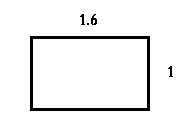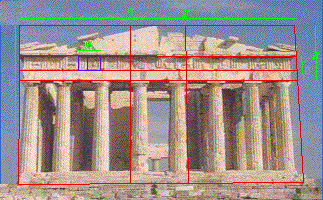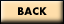# The Golden Rectangle

 The Golden Rectangle refers to rectangles that have a ratio of 1:1.6. This means that if the width of a rectangle is 1 foot long, the length will be 1.6 feet long. The number 1.6 is sometimes referred to as "phi", and it looks like the letter o with a line through it (ø). The Golden Rectangle (or Golden Ratio or Golden Mean or Golden Section) appears in a lot of ancient Greek architecture and has been analyzed extensively by the famous mathematician Fibonacci, as it is related to the number sequence that bears his name. (The Fibonacci number series starts with 0, 1, 1, 2, 3, 5, 8, 13 and so on, with the each number in the series being the sum of the previous two numbers. Dividing any number in the series by the preceding number, such as 8 divided by 5, equals phi) The ratio of 1:1.6 is said to be "pleasing to the eye." Where else can you find examples of the Golden Ratio in your classroom?This is a picture of the Parthenon with some "Golden Rectangles" identified. From The Parthenon and Greek ArchitectureMath-Kitecture Home